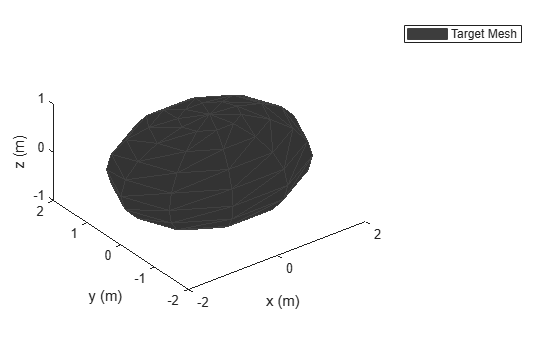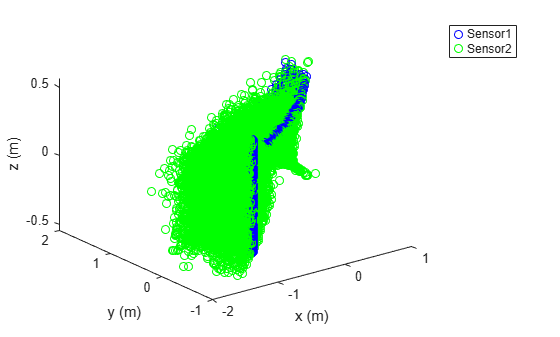# lidarDetect

Report point cloud detections from all lidar sensor in `trackingScenario`

Since R2020b

## Syntax

``pointCloulds = lidarDetect(scene)``
``[pointClouds,configs] = lidarDetect(scene)``
``[pointClouds,configs, clusters] = lidarDetect(___)``

## Description

example

````pointCloulds = lidarDetect(scene)` reports point cloud detections from all `monostaticLidarSensor` objects mounted on every platform in the `trackingScenario`, `scene`. ```
````[pointClouds,configs] = lidarDetect(scene)` also returns the configurations of the sensors, `configs`, in the tracking scenario.```
````[pointClouds,configs, clusters] = lidarDetect(___)` also returns `clusters`, the cluster labels for each point in the point cloud detections.```

## Examples

collapse all

Create a tracking scenario.

```sc = trackingScenario; rng(2020) % for repeatable results```

Add two platforms to the tracking scenario.

```plat1 = platform(sc); plat2 = platform(sc);```

Add a target platform to the tracking scenario.

`target = platform(sc);`

Define a simple waypoint trajectory for the target.

```traj = waypointTrajectory("Waypoints",[1 1 1; 2 2 2],"TimeOfArrival",[0,1]); target.Trajectory = traj;```

Define a sphere mesh for the target.

```target.Mesh = extendedObjectMesh("Sphere"); target.Dimensions = struct("Length",4,"Width",3,"Height",2,"OriginOffset",[0 0 0]);```

Show the mesh of the target.

```figure() show(target.Mesh); legend("Target Mesh") xlabel('x (m)'); ylabel('y (m)'); zlabel('z (m)');```Create two lidar sensors with different range accuracy. Mount them on the two platforms.

```sensor1 = monostaticLidarSensor(1,"RangeAccuracy",0.01); sensor2 = monostaticLidarSensor(2,"RangeAccuracy",0.2); plat1.Sensors = {sensor1}; plat2.Sensors = {sensor2};```

Generate detections from the two lidar sensor using `lidarDetect`.

`[pointClouds,configs,clusters] = lidarDetect(sc);`

Visualize the results.

```cloud1 = pointClouds{1}; cloud2 = pointClouds{2}; figure() plot3(cloud1(:,1),cloud1(:,2),cloud1(:,3),'bo') hold on plot3(cloud2(:,1),cloud2(:,2),cloud2(:,3),'go') legend('Sensor1','Sensor2') xlabel('x (m)'); ylabel('y (m)'); zlabel('z (m)')```## Input Arguments

collapse all

Tracking scenario, specified as a `trackingScenario` object.

## Output Arguments

collapse all

Point cloud detections generated by the sensors, returned as a K-element cell array. K is the number of `monostaticLidarSensor` objects in the tracking scenario, `scene`. Each cell element is an array representing the point cloud generated by the corresponding sensor. The dimension of the array is determined by the `HasOrganizedOuput` property of the sensor.

• When this property is set as `true`, the cell element is returned an N-by-M-by-3 array of scalars, where N is the number of elevation channels, and M is the number of azimuth channels.

• When this property is set as `false`, the cell element is returned as an P-by-3 matrix of scalars, where P is the product of the numbers of elevation and azimuth channels.

The coordinate frame in which the point cloud locations are reported is determined by the `DetectionCoordinates` property of the sensor.

Current sensor configurations, returned as a K-element array of structures. K is the number of `monostaticLidarSensor` objects in the tracking scenario, `scene`. Each structure has these fields:

 Field Description `SensorIndex` Unique sensor index, returned as a positive integer. `IsValidTime` Valid detection time, returned as `true` or `false`. `IsValidTime` is `false` when detection updates are requested between update intervals specified by the update rate. `IsScanDone` `IsScanDone` is `true` when the sensor has completed a scan. `FieldOfView` Field of view of the sensor, returned as a 2-by-2 matrix of positive real values. The first row elements are the lower and upper azimuth limits; the second row elements are the lower and upper elevation limits. `MeasurementParameters` Sensor measurement parameters, returned as an array of structures containing the coordinate frame transforms needed to transform positions and velocities in the top-level frame to the current sensor frame.

Data Types: `struct`

Cluster labels of points in the `pointClouds` output, returned as a K-element cell array. K is the number of `monostaticLidarSensor` in the tracking scenario, `scene`. Each cell element is an array representing cluster labels of points in the point cloud generated by the corresponding sensor. The dimension of the array is determined by the `HasOrganizedOuput` of the sensor.

• When this property is set as `true`, the cell element is returned as an N-by-M-by-2 array of scalars, where N is the number of elevation channels, and M is the number of azimuth channels. On the third dimension, the first element represents the `PlatformID` of the target generating the point, and the second element represents the `ClassID` of the target.

• When this property is set as `false`, the cell element is returned as a P-by-2 matrix of scalars, where P is the product of the numbers of elevation and azimuth channels. For each column of the matrix, the first element represents the `PlatformID` of the target generating the point whereas the second element represents the `ClassID` of the target.

## Version History

Introduced in R2020b